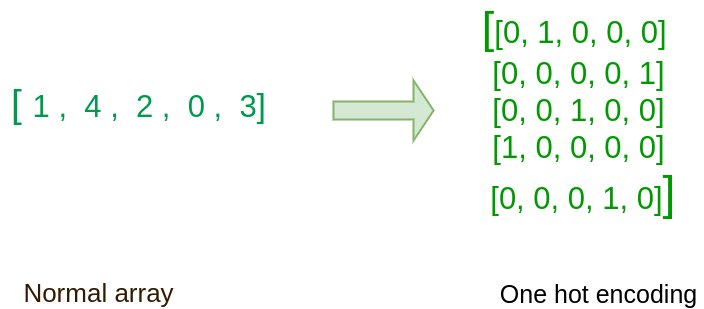# One Hot Encoding using Tensorflow

• Difficulty Level : Medium
• Last Updated : 02 Sep, 2020

In this post, we will be seeing how to initialize a vector in TensorFlow with all zeros or ones. The function you will be calling is `tf.ones()`. To initialize with zeros you could use `tf.zeros()` instead. These functions take in a shape and return an array full of zeros and ones accordingly.

Code:

Attention reader! Don’t stop learning now. Get hold of all the important Machine Learning Concepts with the Machine Learning Foundation Course at a student-friendly price and become industry ready.

 `import` `tensorflow as tf`` ` `ones_matrix ``=` `tf.ones([``2``, ``3``])``sess ``=` `tf.Session()``ones ``=` `sess.run(ones_matrix)``sess.close()`` ` `print``(ones)`

Output:

`[[1. 1. 1.]  [1. 1. 1.]]`

Using One Hot Encoding:
Many times in deep learning and general vector computations you will have a y vector with numbers ranging from 0 to C-1 and you want to do the following conversion. If C is for example 5, then you might have the following y vector which you will need to convert as follows:One Hot Encoding Example

This can be done as follows:

Parameters passed to the function:

indices: A Tensor of indices.
depth: A scalar defining the depth of the one hot dimension.
on_value: A scalar defining the value to fill in output when indices[j] = i. (default : 1)
off_value: A scalar defining the value to fill in output when indices[j] != i. (default : 0)
axis: The axis to fill (default : -1, a new inner-most axis).
dtype: The data type of the output tensor.
name: A name for the operation (optional).

Code:

 `indices ``=` `[``1``, ``4``, ``2``, ``0``, ``3``]``C ``=` `tf.constant(``5``, name ``=` `"C"``)``     ` `one_hot_matrix ``=` `tf.one_hot(``    ``indices, C, on_value ``=` `1.0``, off_value ``=` `0.0``, axis ``=``-``1``)`` ` `sess ``=` `tf.Session()`` ` `one_hot ``=` `sess.run(one_hot_matrix)`` ` `sess.close()`` ` `# output is of dimension 5 x 5``print``(one_hot)`

Output:

```[[0.0, 1.0, 0.0, 0.0, 0.0 ]

[0.0, 0.0, 0.0, 0.0, 1.0]

[0.0, 0.0, 1.0, 0.0, 0.0]

[1.0, 0.0, 0.0, 0.0, 0.0]

[0.0, 0.0, 0.0, 1.0, 0.0]]```

Feel free to change values and see the result.

Code:

 `indices ``=` `[[``0``, ``2``], [``1``, ``-``1``]]``C ``=` `tf.constant(``5``, name ``=` `"C"``)``     ` `one_hot_matrix ``=` `tf.one_hot(``    ``indices, C, on_value ``=` `1.0``, off_value ``=` `0.0``, axis ``=``-``1``)`` ` `sess ``=` `tf.Session()`` ` `one_hot ``=` `sess.run(one_hot_matrix)`` ` `sess.close()``     ` `# output is of dimension 2 x 2 x 3``print``(one_hot) `

Output :

```[[[1.0, 0.0, 0.0],

[0.0, 0.0, 1.0]],

[[0.0, 1.0, 0.0],

[0.0, 0.0, 0.0]]] ```

My Personal Notes arrow_drop_up